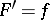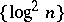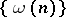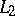# Metric theory of functions

Jump to: navigation, search

The branch of the theory of functions of a real variable (cf. Functions of a real variable, theory of) in which properties of functions are studied on the basis of the idea of the measure of a set.

The research of many 19th century mathematicians created a new mathematical discipline — the theory of functions of a real variable. At the end of the 19th century there was a clear crystallization of certain problems which required solution: the problem of the measure of a set, the length of curves and the area of surfaces, the representation of functions by series (in particular, by trigonometric series), the primitive and the integral, relations between integration and differentiation, term-by-term integration of series, etc. The solution of these problems had a general mathematical significance; the most prominent mathematicians worked in this direction, which, in particular, explained the vigorous development of the metric theory of functions in the first third of the 20th century. The foundations of this theory of functions were laid by E. Borel, R. Baire, H. Lebesgue, and others.

In 1902 Lebesgue introduced the extraordinarily important idea of the measure of a set (Lebesgue measure). On the basis of this idea he created a theory of the integral (Lebesgue integral). These two fundamental ideas — measure and integral — formed the foundation of the metric theory of functions, which is concerned with the study of properties of functions, derivatives, integrals, series of functions (in particular, trigonometric and general orthogonal series), areas of surfaces, etc.

Many basic properties of the Lebesgue measure and integral were established at the beginning of the 20th century by Lebesgue himself (countable additivity of the measure and the integral, passage to the limit under the integral sign, differentiation of an indefinite integral, etc.). In addition, Lebesgue gave numerous applications of his research to various questions in mathematical analysis (area of surfaces, various properties of trigonometric series, singular integrals, etc.). G. Vitali (1904) independently discovered a measure identical to the Lebesgue measure, and, somewhat later, W.H. Young (1905) also constructed an integral and a measure equivalent to the Lebesgue integral and measure. However, they did not develop their theories and, in this period, made no significant applications of them. The origin of the development of the metric theory of functions in Russia must be associated with the beginning of the 20th century. However, the first important results in this area were obtained by Russian mathematicians in the second decade of the 20th century (D.F. Egorov, N.N. Luzin), when a new important centre of research into the metric theory of functions was formed. The founder and leader of the school of the metric theory of functions in the USSR was Luzin.

The development of the metric theory of functions can be characterized by two major directions. The first direction was to study on the basis of the measure of a set and the Lebesgue integral, and also their generalizations, both general properties of functions, integrals, trigonometric series, orthogonal series, etc., and more concrete subtle properties of them, the development and study of which by classical analytic methods was almost unattainable. This direction is, precisely, the metric theory of functions. The second, no less important direction, was the penetration of methods of the metric theory of functions into other divisions of mathematics, and also the creation, based on its ideas, of other new areas of mathematics which, in their turn, had a stimulating effect on the theory of functions itself.

On the basis of the metric theory of functions a detailed study of boundary properties of analytic functions was initiated and the metric theory of numbers, whose methods are inseparably connected with the metric theory of functions, was created. The influence of the theory of functions on the creation of functional analysis was great.

Below, certain typical results from the metric theory of functions are given, each of which signified the solution of some central question and entailed numerous subsequent investigations. Thus, in 1911, Egorov proved that every convergent sequence of measurable functions is uniformly convergent if some set of arbitrarily small measure is neglected (see Egorov theorem). Luzin (1912) established that every measurable function becomes continuous if some set of arbitrarily small measure is neglected (see Luzin-property). These two results are difficult to overestimate since, on the one hand, they have shown a definite interrelation between basic ideas of the metric theory of functions and classical analysis, and, on the other hand, they are the foundation on which much research in the theory of functions has often been conducted.

Luzin proved (1915) the existence of primitives in the class of measurable functions. Namely, he proved that for every finite measurable functionthere is a continuous functionsuch thatalmost-everywhere. On the basis of this result he solved the Dirichlet problem in the class of measurable functions. This was the beginning of an active development of the Moscow school concerning the theory of functions of a complex variable and, in particular, the beginning of the study of boundary properties of analytic functions.

The Lebesgue integral does not solve the problem of finding a primitivefrom the finite exact derivative. This problem was solved by A. Denjoy (1912) on the basis of a special integral (the narrow Denjoy integral) which naturally generalizes the Lebesgue integral and does not contradict it (see Denjoy integral). In 1916 Denjoy and A.Ya. Khinchin constructed an even more general integral, named the Denjoy integral in the wide sense; it is related to approximate differentiability.

At the beginning of the-rd decade of the 20th century D.E. Men'shov and H. Rademacher established that the sequenceis a Weyl multiplier for the almost-everywhere convergence of series in any orthogonal system. In addition (and this is particularly important and fundamental), Men'shov proved (1923) that the above result is not true if the sequenceis replaced by any sequencewithas. These results became the foundation on which various investigations into the theory of convergence and summability of orthogonal series have been and still are being built.

In 1926 Kolmogorov constructed an example of an everywhere-divergent trigonometric Fourier series of a summable function. However, the problem of almost-everywhere convergence of the trigonometric Fourier series of a function in, proposed by Luzin in 1914, remained unsolved for a long time. A positive solution to this problem was given by L. Carleson only in 1966 (see Carleson theorem). P. du Bois-Reymond, Lebesgue and Ch. de la Vallée-Poussin solved the problem of the recovery of the coefficients of a trigonometric series which converges to a summable function. In the 1940's Denjoy constructed an integral by which the problem of the recovery of coefficients in a sum of an arbitrary everywhere-convergent trigonometric series could be solved. At the same time Men'shov proved that every finite measurable function is representable by some almost-everywhere convergent trigonometric series.

G. Cantor, Young, N.K. Bari, etc., have laid the foundations of the theory of uniqueness of trigonometric series.